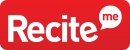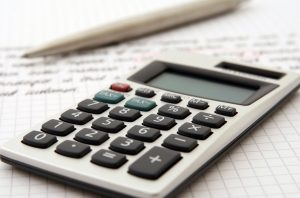A teacher writes, “I have a new student who transferred from a public charter school. She has an IEP accommodation for a calculator use for anything involving math calculations. But I’m wondering whether providing a calculator is changing standards? Is she learning how to use a calculator rather than learning how to do math? Where do we draw the line?”

I’m glad you asked this question. I think more teacher and parents ask themselves this question than you may realize.

Dyscalculia is one of the least recognized “specific learning disabilities.” In the past ten years, there’s been a revolution in our understanding of the scientific basis of dyscalculia, but in day-to-day practice, there’s remarkably little in the way of practical guidance for both teachers and students.

For teachers, the goal for accommodations and modifications should be to optimize student learning. There are a variety of reasons why student may struggle with math facts fluency, but still also be able to succeed at the highest levels of mathematics.

What is a mistake is making mental math or pencil-dependent math calculations the narrow gate through which all math problem solving must go through. This would be a grave mistake, because there are talented future scientists and even mathematicians who struggle with remembering basic math facts.

Use of a calculator is not ‘cheating’ for a student with dyscalculia.

A calculator allows dyscalculic students to access higher levels of math problem solving. Students must still recognize the correct approaches to solving problems and follow appropriate steps to arrive at final answers. Using a free-standing calculator or calculator on a phone is a life skill for this student.

I’m not sure what age your student is, but many junior high and high schools let students use calculators for all math and science work. For students in elementary school, it is possible that some students may just need more time (some may need A LOT more time) to master their facts and perform pencil and paper calculations. If that is the case, then allowing them to work ‘open book’ with a math facts table while solving problems could still help a student progress with other types of math while also receiving ongoing positive reinforcement about number facts.

I’m not sure what age your student is, but many junior high and high schools let students use calculators for all math and science work. For students in elementary school, it is possible that some students may just need more time (some may need A LOT more time) to master their facts and perform pencil and paper calculations. If that is the case, then allowing them to work ‘open book’ with a math facts table while solving problems could still help a student progress with other types of math while also receiving ongoing positive reinforcement about number facts.